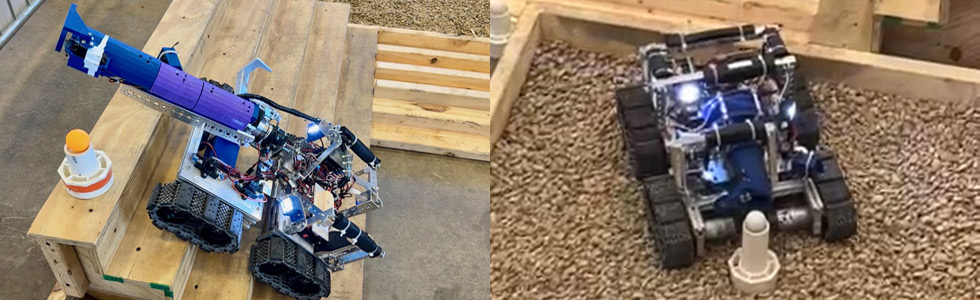GEAR - Geauga Engineering and Robotics## Introductory Lessons for First Year Seniors 2021

Lesson 1 - Electricity and Electronics for Robotics - This is the first lesson for first year senior members.

READ THIS BEFORE STARTING LESSON 2

Lesson 2 - Electrical and Electronic Circuits This lesson covers the basic information you need to learn about circuits and electronic parts.

## Line following robot

Data sheet for red LED used in this lesson.

Data sheet for switch used in this lesson.

Data sheet for photoresistors used in this lesson.

Data sheet for R3 potentiometer used in this lesson. This data sheet covers several types. The one we are using is PV36W with drawings on pages 67 and 69 of the data sheet. This is a 25 turn pot, which means you must turn the screw 25 turns to achieve the full range of resistance adjustments possible.

Resistor exercise This exercise is designed to help members understand the photoresistor circuit.

Data sheet for LM393 IC chip used in this lesson.

Data sheet for green LED used in this lesson.

Data sheet for yellow LED used in this lesson.

Data sheet for transistors used in this lesson.

Lesson 5, DC Motors This is the last lesson for breadboard wiring. After this you will learn to solder and start building your real robot!

Data sheet for Schottky diode used in this lesson.

Data sheet for motor used in this lesson.

Another data sheet for motor used in this lesson.

And a third data sheet for motor used in this lesson.

Manufacture of a magnet Here is a nice video covering a manufacturing process for permanent magnets

Schematic of breadboard circuits (except power circuit)

The lessons above will prepare our members to build Sandwich, a line following robot, found in the book "Robot Building for Beginners" by David Cook.

Parts List This document provides the parts list for the robot.

Wheel for robot This is a mechanical drawing for 3D printed wheel. Use a square cross section O-ring for tire (McMaster-Carr part number 4061T251. McMaster-Carr)

Mechanical Drawings of Robot Frame

Top View

Side View

Back View

Front View

Isometric View

Another Isometric View

If you would like to see this robot in action, there are several videos on YouTube.
Here is one
Here is a web page about the robot.